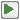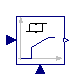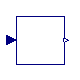## Buildings.Controls.Continuous

Package with models for discrete time controls

### Information

Extends from Modelica.Icons.Package (Icon for standard packages).

### Package Content

Name DescriptionLimPID P, PI, PD, and PID controller with limited output, anti-windup compensation and setpoint weightingNumberOfRequests Outputs the number of signals that are above/below a certain thresholdOffTimer Records the time since the input changed to falsePIDHysteresis PID controller with anti-windup, output limiter and output hysteresisPIDHysteresisTimer PID controller with anti-windup, hysteresis and timer to prevent short cyclingSignalRanker Ranks output signals such that y[i] >= y[i+1]Examples Collection of models that illustrate model use and test modelsValidation Collection of validation models

##Buildings.Controls.Continuous.LimPID

P, PI, PD, and PID controller with limited output, anti-windup compensation and setpoint weighting### Information

PID controller in the standard form

y = k   ( e(t) + 1 ⁄ Ti   ∫ e(s) ds + Td de(t)⁄dt ),

where y is the control signal, e(t) = us - um is the control error, with us being the set point and um being the measured quantity, k is the gain, Ti is the time constant of the integral term and Td is the time constant of the derivative term.

Note that the units of k are the inverse of the units of the control error, while the units of Ti and Td are seconds.

For detailed treatment of integrator anti-windup, set-point weights and output limitation, see Modelica.Blocks.Continuous.LimPID.

#### Options

This controller can be configured as follows.
##### P, PI, PD, or PID action

Through the parameter `controllerType`, the controller can be configured as P, PI, PD or PID controller. The default configuration is PI.

##### Direct or reverse acting

Through the parameter `reverseActing`, the controller can be configured to be reverse or direct acting. The above standard form is reverse acting, which is the default configuration. For a reverse acting controller, for a constant set point, an increase in measurement signal `u_m` decreases the control output signal `y` (Montgomery and McDowall, 2008). Thus,

• for a heating coil with a two-way valve, leave `reverseActing = true`, but
• for a cooling coil with a two-way valve, set `reverseActing = false`.
##### Reset of the controller output

The controller can be configured to enable an input port that allows resetting the controller output. The controller output can be reset as follows:

• If `reset = Buildings.Types.Reset.Disabled`, which is the default, then the controller output is never reset.
• If `reset = Buildings.Types.Reset.Parameter`, then a boolean input signal `trigger` is enabled. Whenever the value of this input changes from `false` to `true`, the controller output is reset by setting `y` to the value of the parameter `y_reset`.
• If `reset = Buildings.Types.Reset.Input`, then a boolean input signal `trigger` and a real input signal `y_reset_in` are enabled. Whenever the value of `trigger` changes from `false` to `true`, the controller output is reset by setting the value of `y` to `y_reset_in`.

Note that this controller implements an integrator anti-windup. Therefore, for most applications, keeping the default setting of `reset = Buildings.Types.Reset.Disabled` is sufficient. However, if the controller is used in conjuction with equipment that is being switched on, better control performance may be achieved by resetting the controller output when the equipment is switched on. This is in particular the case in situations where the equipment control input should continuously increase as the equipment is switched on, such as a light dimmer that may slowly increase the luminance, or a variable speed drive of a motor that should continuously increase the speed.

#### References

R. Montgomery and R. McDowall (2008). "Fundamentals of HVAC Control Systems." American Society of Heating Refrigerating and Air-Conditioning Engineers Inc. Atlanta, GA.

Extends from Modelica.Blocks.Interfaces.SVcontrol (Single-Variable continuous controller).

### Parameters

TypeNameDefaultDescription
SimpleControllercontrollerTypeModelica.Blocks.Types.Simple...Type of controller
Realk1Gain of controller
TimeTi0.5Time constant of Integrator block [s]
TimeTd0.1Time constant of Derivative block [s]
RealyMax1Upper limit of output
RealyMin0Lower limit of output
Realwp1Set-point weight for Proportional block (0..1)
Realwd0Set-point weight for Derivative block (0..1)
RealNi0.9Ni*Ti is time constant of anti-windup compensation
RealNd10The higher Nd, the more ideal the derivative block
BooleanreverseActingtrueSet to true for reverse acting, or false for direct acting control action
Initialization
InitPIDinitTypeModelica.Blocks.Types.InitPI...Type of initialization (1: no init, 2: steady state, 3: initial state, 4: initial output)
Realxi_start0Initial or guess value value for integrator output (= integrator state)
Realxd_start0Initial or guess value for state of derivative block
Realy_start0Initial value of output
Integrator reset
ResetresetBuildings.Types.Reset.DisabledType of controller output reset
Realy_resetxi_startValue to which the controller output is reset if the boolean trigger has a rising edge, used if reset == Buildings.Types.Reset.Parameter
Booleanstricttrue= true, if strict limits with noEvent(..)

### Connectors

TypeNameDescription
input RealInputu_sConnector of setpoint input signal
input RealInputu_mConnector of measurement input signal
output RealOutputyConnector of actuator output signal
input BooleanInputtriggerResets the controller output when trigger becomes true
input RealInputy_reset_inInput signal for state to which integrator is reset, enabled if reset = Buildings.Types.Reset.Input

##Buildings.Controls.Continuous.NumberOfRequests

Outputs the number of signals that are above/below a certain threshold### Information

Block that outputs the number of inputs that exceed a threshold. The parameter `kind` is used to determine the kind of the inequality. The table below shows the allowed settings.

Value of parameter `kind` Output signal incremented by 1 for each i ∈ {1, ..., nin} if
0 `u[i] > threShold`
1 `u[i] ≥ threShold`
2 `u[i] ≤ threShold`
3 `u[i] < threShold`

This model may be used to check how many rooms exceed a temperature threshold.

Extends from Modelica.Blocks.Icons.Block (Basic graphical layout of input/output block).

### Parameters

TypeNameDefaultDescription
Integernin Number of inputs
RealthreShold0Threshold
Integerkind Set to 0 for u>threShold, to 1 for >=, to 2 for <= or to 3 for <

### Connectors

TypeNameDescription
output IntegerOutputyNumber of input signals that violate the threshold
input RealInputu[nin]Input signals

### Modelica definition

block NumberOfRequests "Outputs the number of signals that are above/below a certain threshold" extends Modelica.Blocks.Icons.Block; parameter Integer nin "Number of inputs"; parameter Real threShold = 0 "Threshold"; parameter Integer kind "Set to 0 for u>threShold, to 1 for >=, to 2 for <= or to 3 for <"; Modelica.Blocks.Interfaces.IntegerOutput y "Number of input signals that violate the threshold"; Modelica.Blocks.Interfaces.RealInput u[nin] "Input signals"; algorithm y := 0; for i in 1:nin loop if kind == 0 then if u[i] > threShold then y := y+1; end if; end if; if kind == 1 then if u[i] >= threShold then y := y+1; end if; end if; if kind == 2 then if u[i] <= threShold then y := y+1; end if; end if; if kind == 3 then if u[i] < threShold then y := y+1; end if; end if; end for; end NumberOfRequests;

##Buildings.Controls.Continuous.OffTimer

Records the time since the input changed to false### Information

Timer that starts at the initial time with a value of 0, and gets reset each time the input signal switches to `false`.

For example, if the simulation starts at t = 10 and at t=11, the input becomes `false`, then the timer outputs y=t-10 for t < 11, and y=t-11 afterwards, unless the input becomes false again.

Extends from Modelica.Blocks.Icons.PartialBooleanBlock (Basic graphical layout of logical block).

### Connectors

TypeNameDescription
input BooleanInputuConnector of Boolean input signal
output RealOutputyConnector of Real output signal

### Modelica definition

model OffTimer "Records the time since the input changed to false" extends Modelica.Blocks.Icons.PartialBooleanBlock; Modelica.Blocks.Interfaces.BooleanInput u "Connector of Boolean input signal"; Modelica.Blocks.Interfaces.RealOutput y "Connector of Real output signal"; protected discrete Modelica.SIunits.Time entryTime "Time instant when u became true"; initial equation pre(entryTime) = time; equation when (not u) then entryTime = time; end when; y = time - entryTime; end OffTimer;

##Buildings.Controls.Continuous.PIDHysteresis

PID controller with anti-windup, output limiter and output hysteresis### Information

Block of a controller for set point tracking with a hysteresis element that switches the controller on and off.

If the controller is off, and the control error becomes larger than `eOn`, then the controller switches to on and remains on until the control error is smaller than `eOff`. When the controller is on, the set point tracking can be done using a P-, PI-, or PID-controller. In its off-mode, the control output is zero. Thus, the parameters `yMin` and `yMax` are used to constrain the output of the controller during its on mode only. This can be used, for example, to modulate a device between 0.3 and 1.0, and switch it to off when the control error is small enough.

Extends from Modelica.Blocks.Interfaces.SVcontrol (Single-Variable continuous controller).

### Parameters

TypeNameDefaultDescription
Hysteresis
RealeOn1if off and control error > eOn, switch to set point tracking
RealeOff-eOnif on and control error < eOff, set y=0
Booleanpre_y_startfalseValue of hysteresis output at initial time
Set point tracking
SimpleControllercontrollerTypeModelica.Blocks.Types.Simple...Type of controller
Realk1Gain of controller
TimeTi Time constant of Integrator block [s]
TimeTd Time constant of Derivative block [s]
RealyMax1Upper limit of output
RealyMin0Lower limit of output
Realwp1Set-point weight for Proportional block (0..1)
Realwd0Set-point weight for Derivative block (0..1)
RealNi0.9Ni*Ti is time constant of anti-windup compensation
RealNd10The higher Nd, the more ideal the derivative block
BooleanreverseActingtrueSet to true for reverse acting, or false for direct acting control action
Initialization
InitPIDinitTypeModelica.Blocks.Types.InitPI...Type of initialization (1: no init, 2: steady state, 3: initial state, 4: initial output)
Realxi_start0Initial or guess value value for integrator output (= integrator state)
Realxd_start0Initial or guess value for state of derivative block
Realy_start0Initial value of output
Booleanstricttrue= true, if strict limits with noEvent(..)

### Connectors

TypeNameDescription
input RealInputu_sConnector of setpoint input signal
input RealInputu_mConnector of measurement input signal
output RealOutputyConnector of actuator output signal

### Modelica definition

model PIDHysteresis "PID controller with anti-windup, output limiter and output hysteresis" extends Modelica.Blocks.Interfaces.SVcontrol; parameter Real eOn = 1 "if off and control error > eOn, switch to set point tracking"; parameter Real eOff = -eOn "if on and control error < eOff, set y=0"; parameter Boolean pre_y_start=false "Value of hysteresis output at initial time"; parameter Modelica.Blocks.Types.SimpleController controllerType=Modelica.Blocks.Types.SimpleController.PI "Type of controller"; parameter Real k=1 "Gain of controller"; parameter Modelica.SIunits.Time Ti "Time constant of Integrator block"; parameter Modelica.SIunits.Time Td "Time constant of Derivative block"; parameter Real yMax=1 "Upper limit of output"; parameter Real yMin=0 "Lower limit of output"; parameter Real wp=1 "Set-point weight for Proportional block (0..1)"; parameter Real wd=0 "Set-point weight for Derivative block (0..1)"; parameter Real Ni=0.9 "Ni*Ti is time constant of anti-windup compensation"; parameter Real Nd=10 "The higher Nd, the more ideal the derivative block"; parameter Boolean reverseActing = true "Set to true for reverse acting, or false for direct acting control action"; parameter Modelica.Blocks.Types.InitPID initType=Modelica.Blocks.Types.InitPID.DoNotUse_InitialIntegratorState "Type of initialization (1: no init, 2: steady state, 3: initial state, 4: initial output)"; parameter Real xi_start=0 "Initial or guess value value for integrator output (= integrator state)"; parameter Real xd_start=0 "Initial or guess value for state of derivative block"; parameter Real y_start=0 "Initial value of output"; parameter Boolean strict=true "= true, if strict limits with noEvent(..)"; LimPID PID( final controllerType=controllerType, final k=k, final Ti=Ti, final yMax=yMax, final yMin=yMin, final wp=wp, final wd=wd, final Ni=Ni, final Nd=Nd, final initType=initType, final xi_start=xi_start, final xd_start=xd_start, final y_start=y_start, final Td=Td, final reverseActing=reverseActing, final strict=strict) "Controller for room temperature"; Modelica.Blocks.Logical.Hysteresis hys( final pre_y_start=pre_y_start, final uLow=eOff, final uHigh=eOn) "Hysteresis element to switch controller on and off"; Modelica.Blocks.Math.Feedback feeBac; protected Modelica.Blocks.Logical.Switch swi; Modelica.Blocks.Sources.Constant zer(final k=0) "Zero signal"; Modelica.Blocks.Logical.Switch swi1; equation assert(eOff < eOn, "Wrong controller parameters. Require eOff < eOn."); connect(zer.y, swi.u3); connect(swi.y, y); connect(u_m, PID.u_m); connect(hys.y, swi.u2); connect(PID.y, swi.u1); connect(u_s, feeBac.u1); connect(u_m, feeBac.u2); connect(feeBac.y, hys.u); connect(u_s, swi1.u1); connect(hys.y, swi1.u2); connect(u_m, swi1.u3); connect(swi1.y, PID.u_s); end PIDHysteresis;

##Buildings.Controls.Continuous.PIDHysteresisTimer

PID controller with anti-windup, hysteresis and timer to prevent short cycling### Information

Block of a controller for set point tracking with a hysteresis element that switches the controller on and off, and a timer that prevents the controller to short cycle.

The controller is similar to Buildings.Controls.Continuous.PIDHysteresis but in addition, it has a timer that prevents the controller from switching to on too fast. When the controller switches off, the timer starts and avoids the controller from switching on until `minOffTime` seconds elapsed.

Extends from Modelica.Blocks.Interfaces.SVcontrol (Single-Variable continuous controller).

### Parameters

TypeNameDefaultDescription
On/off controller
RealminOffTime600Minimum time that devices needs to be off before it can run again
RealeOn1if off and control error > eOn, switch to set point tracking
RealeOff-eOnif on and control error < eOff, set y=0
Booleanpre_y_startfalseValue of hysteresis output at initial time
Set point tracking
SimpleControllercontrollerTypeModelica.Blocks.Types.Simple...Type of controller
Realk1Gain of controller
TimeTi Time constant of Integrator block [s]
TimeTd Time constant of Derivative block [s]
RealyMax1Upper limit of modulating output
RealyMin0.3Lower limit of modulating output (before switch to 0)
Realwp1Set-point weight for Proportional block (0..1)
Realwd0Set-point weight for Derivative block (0..1)
RealNi0.9Ni*Ti is time constant of anti-windup compensation
RealNd10The higher Nd, the more ideal the derivative block
BooleanreverseActingtrueSet to true for reverse acting, or false for direct acting control action
Initialization
InitPIDinitTypeModelica.Blocks.Types.InitPI...Type of initialization (1: no init, 2: steady state, 3: initial state, 4: initial output)
Realxi_start0Initial or guess value value for integrator output (= integrator state)
Realxd_start0Initial or guess value for state of derivative block
Realy_start0Initial value of output
Booleanstricttrue= true, if strict limits with noEvent(..)

### Connectors

TypeNameDescription
input RealInputu_sConnector of setpoint input signal
input RealInputu_mConnector of measurement input signal
output RealOutputyConnector of actuator output signal
output RealOutputtOnTime since boiler switched on
output RealOutputtOffTime since boiler switched off
output BooleanOutputonOutputs true if boiler is on

### Modelica definition

model PIDHysteresisTimer "PID controller with anti-windup, hysteresis and timer to prevent short cycling" extends Modelica.Blocks.Interfaces.SVcontrol; parameter Real minOffTime=600 "Minimum time that devices needs to be off before it can run again"; parameter Real eOn = 1 "if off and control error > eOn, switch to set point tracking"; parameter Real eOff = -eOn "if on and control error < eOff, set y=0"; parameter Boolean pre_y_start=false "Value of hysteresis output at initial time"; parameter Modelica.Blocks.Types.SimpleController controllerType=Modelica.Blocks.Types.SimpleController.PI "Type of controller"; parameter Real k=1 "Gain of controller"; parameter Modelica.SIunits.Time Ti "Time constant of Integrator block"; parameter Modelica.SIunits.Time Td "Time constant of Derivative block"; parameter Real yMax=1 "Upper limit of modulating output"; parameter Real yMin=0.3 "Lower limit of modulating output (before switch to 0)"; parameter Real wp=1 "Set-point weight for Proportional block (0..1)"; parameter Real wd=0 "Set-point weight for Derivative block (0..1)"; parameter Real Ni=0.9 "Ni*Ti is time constant of anti-windup compensation"; parameter Real Nd=10 "The higher Nd, the more ideal the derivative block"; parameter Boolean reverseActing = true "Set to true for reverse acting, or false for direct acting control action"; parameter Modelica.Blocks.Types.InitPID initType=Modelica.Blocks.Types.InitPID.DoNotUse_InitialIntegratorState "Type of initialization (1: no init, 2: steady state, 3: initial state, 4: initial output)"; parameter Real xi_start=0 "Initial or guess value value for integrator output (= integrator state)"; parameter Real xd_start=0 "Initial or guess value for state of derivative block"; parameter Real y_start=0 "Initial value of output"; parameter Boolean strict=true "= true, if strict limits with noEvent(..)"; Modelica.Blocks.Interfaces.RealOutput tOn "Time since boiler switched on"; Modelica.Blocks.Interfaces.RealOutput tOff "Time since boiler switched off"; LimPID con( final controllerType=controllerType, final k=k, final Ti=Ti, final Td=Td, final wp=wp, final wd=wd, final Ni=Ni, final Nd=Nd, final initType=initType, final xi_start=xi_start, final xd_start=xd_start, final y_start=y_start, final yMin=yMin, final yMax=yMax, final reverseActing=reverseActing, final strict=strict) "Controller to track setpoint"; OffTimer offHys; Modelica.Blocks.Logical.Timer onTimer; Modelica.Blocks.Logical.Timer offTimer; Modelica.Blocks.Interfaces.BooleanOutput on "Outputs true if boiler is on"; Modelica.Blocks.Math.Feedback feeBac; Modelica.Blocks.Logical.Hysteresis hys( final pre_y_start=pre_y_start, final uLow=eOff, final uHigh=eOn) "Hysteresis element to switch controller on and off"; protected Modelica.Blocks.Sources.Constant zer(final k=0) "Zero signal"; Modelica.Blocks.Logical.Switch switch2; Modelica.Blocks.Logical.GreaterEqualThreshold greaterEqualThreshold(threshold= minOffTime); Modelica.Blocks.Logical.And and3; Modelica.Blocks.Logical.Not not1; Modelica.Blocks.Logical.Switch switch1; equation connect(u_m, con.u_m); connect(zer.y,switch2. u3); connect(switch2.y, y); connect(and3.y,switch2. u2); connect(greaterEqualThreshold.y, and3.u1); connect(offHys.y, greaterEqualThreshold.u); connect(con.y,switch2. u1); connect(onTimer.y, tOn); connect(offTimer.y, tOff); connect(and3.y, on); connect(and3.y, onTimer.u); connect(and3.y, not1.u); connect(not1.y, offTimer.u); connect(and3.y, switch1.u2); connect(u_s, switch1.u1); connect(u_m, switch1.u3); connect(switch1.y, con.u_s); connect(u_s, feeBac.u1); connect(u_m, feeBac.u2); connect(feeBac.y, hys.u); connect(hys.y, offHys.u); connect(hys.y, and3.u2); end PIDHysteresisTimer;

##Buildings.Controls.Continuous.SignalRanker

Ranks output signals such that y[i] >= y[i+1]### Information

Block that sorts the input signal `u[:]` such that the output signal satisfies `y[i] >= y[i+1]` for all `i=1, ..., nin-1`.

This block may for example be used in a variable air volume flow controller to access the position of the dampers that are most open.

Extends from Modelica.Blocks.Interfaces.MIMO (Multiple Input Multiple Output continuous control block).

### Parameters

TypeNameDefaultDescription
Integernin1Number of inputs
IntegernoutninNumber of outputs

### Connectors

TypeNameDescription
input RealInputu[nin]Connector of Real input signals
output RealOutputy[nout]Connector of Real output signals

### Modelica definition

block SignalRanker "Ranks output signals such that y[i] >= y[i+1]" extends Modelica.Blocks.Interfaces.MIMO(final nout=nin); protected Real t "Temporary variable"; algorithm y[:] := u[:]; for i in 1:nin loop for j in 1:nin-1 loop if y[j] < y[j+1] then t := y[j+1]; y[j+1] := y[j]; y[j] := t; end if; end for; end for; end SignalRanker;

##Buildings.Controls.Continuous.LimPID.Limiter

Limit the range of a signal### Information

Extends from Modelica.Blocks.Interfaces.SISO (Single Input Single Output continuous control block).

### Parameters

TypeNameDefaultDescription
RealuMax Upper limits of input signals
RealuMin-uMaxLower limits of input signals